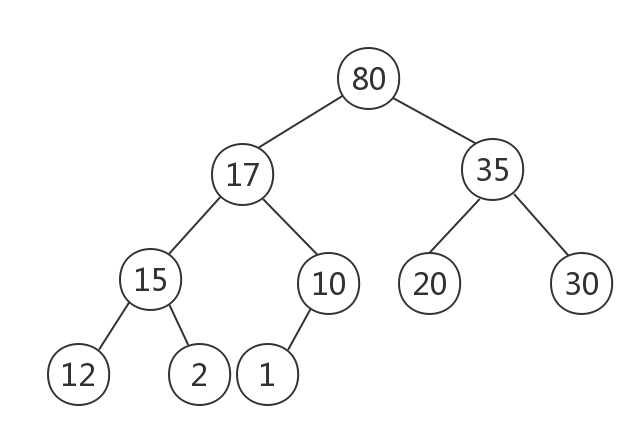• 返回序列中的数 yield n it = filter(_not_divisible(n), it) # 埃氏筛选法,产生筛选后新的序列 list_yc = list() for n in primes(): if n == 17: list_yc.append(n) break list_yc.append(n) print(list_yc) 输出...
代码如下def _odd_iter():  # 构建奇数序列 从3开始n = 1while True:n = n + 2yield ndef _not_divisible(n):return lambda x: x % n > 0def primes():yield 2it = _odd_iter()while True:n = next(it)  # 返回序列中的数yield nit = filter(_not_divisible(n), it) # 埃氏筛选法,产生筛选后新的序列list_yc = list()for n in primes():if n == 17:list_yc.append(n)breaklist_yc.append(n)print(list_yc)输出如下[2, 3, 5, 7, 11, 13, 17]代码分析我不明白代码对别人来说是怎样的难度，我仅说说我自己第一次看到这个代码产生的疑问it = filter(_not_divisible(n), it) 这一行代码中，调用方法 _not_divisible 方法 时，内部的lambda: x: x % n > 0中 x 的值是多少it 是一个 Iterator, 每次next时才会返回一个值(for语句里面是每一次循环都调用一次next), 那序列中的 9 是怎样被 3 筛选掉的呢?第一个问题:这个地方是一个闭包调用，_not_divisible 返回了一个函数，而那个函数作用于 Iterator 中的每一个元素, 即 x 的值是 Iterator 中的每一个值第二个问题:首先追踪 _not_divisible 方法执行的每一步改写 _not_divisible 如下def _not_divisible(n):def lam(x):if n == 3:print('_not_divisible, n == 3', x)elif n == 5:print('_not_divisible, n == 5', x)return x % n > 0return lam输出如下_not_divisible, n == 3 5_not_divisible, n == 3 7_not_divisible, n == 5 7_not_divisible, n == 3 9_not_divisible, n == 3 11_not_divisible, n == 5 11_not_divisible, n == 3 13_not_divisible, n == 5 13_not_divisible, n == 3 15_not_divisible, n == 3 17_not_divisible, n == 5 17埃氏筛选法 可以明显的看到用3，5筛选序列时是交替进行的。可以肯定的是，用3筛选的时候没有一次性筛选所有的数字(事实上也不可能，因为_odd_iter是无限序列)在用数字5筛选的之前，又使用了3筛选。那就是意味着用 3 筛选的方法被挂起了，每当有新数字，该方法会被唤醒且调用。以此类推，每一个数字对应的筛选函数都保存起来了(因为它要作用于序列中的每一个数字)嗯。。。以上想法仅仅是个人折腾代码，调试出来的猜测，尚未向python大神确认。思绪来的快去的也快，偶尔在这里停留
展开全文• 前几天偶尔的有朋友问python怎么判断素数的方法，走网上查了查，总结了python脚本判断一个数是否为素数的几种方法： 1.运用python的数学函数 import math def isPrime(n): if n (2, int(math.sqrt(n)) + 1): if n %...质数又称素数。指在一个大于1的自然数中，除了1和此整数自身外，不能被其他自然数整除的数。素数在数论中有着很重要的地位。比1大但不是素数的数称为合数。1和0既非素数也非合数。质数是与合数相对立的两个概念，二者构成了数论当中最基础的定义之一。基于质数定义的基础之上而建立的问题有很多世界级的难题，如哥德巴赫猜想等。算术基本定理证明每个大于1的正整数都可以写成素数的乘积，并且这种乘积的形式是唯一的。这个定理的重要一点是，将1排斥在素数集合以外。如果1被认为是素数，那么这些严格的阐述就不得不加上一些限制条件。 前几天偶尔的有朋友问python怎么判断素数的方法，走网上查了查，总结了python脚本判断一个数是否为素数的几种方法：1.运用python的数学函数import mathdef isPrime(n):if n <= 1:return Falsefor i in range(2, int(math.sqrt(n)) + 1):if n % i == 0:return Falsereturn True2.单行程序扫描素数from math import sqrtN = 100[ p for p in  range(2, N) if 0 not in [ p% d for d in range(2, int(sqrt(p))+1)] ]运用python的itertools模块from itertools import countdef isPrime(n): www.ddpool.cnif n <= 1:return Falsefor i in count(2):if i * i > n:return Trueif n % i == 0:return False3.不使用模块的两种方法方法1：def isPrime(n):if n <= 1:return Falsei = 2while i*i <= n:if n % i == 0:return Falsei += 1return True方法2：def isPrime(n):if n <= 1:return Falseif n == 2:return Trueif n % 2 == 0:return Falsei = 3while i * i <= n:if n % i == 0:return Falsei += 2return Trueeg：求出20001到40001之间的质数(素数)既然只能被1或者自己整出,那说明只有2次余数为0的时候，代码如下:#!/usr/bin/pythonL1=[]for x in xrange(20001,40001):n = 0for y in xrange(1,x+1):if x % y == 0:n = n + 1if n == 2 :print xL1.append(x)print L1结果如下:2001120021200232002920047200512006320071200892010120107201132011720123201292014320147201492016120173….
展开全文• 向下调整：对于某个结点i，将其与左右子结点中值较大的一个进行比较，如果i的值小于该子节点的值，则将两个结点交换位置；重复上述步骤，直到结点m不再小于左右子结点中值较大的那个或者结点m为叶子结点为止。 ...
最大树（最小树）：每个结点的值都大于（小于）或等于其子节点（如果有的话）的值的树。

最大堆（最小堆）：最大（最小）的完全二叉树

向下调整法：对于某个结点i，将其与左右子结点中值较大的一个进行比较，如果i的值小于该子节点的值，则将两个结点交换位置；重复上述步骤，直到结点m不再小于左右子结点中值较大的那个或者结点m为叶子结点为止。

向上调整法：对于某个结点i，将其与父节点进行比较，如果i的值大于父节点的值，则交换两个结点的位置，重复上述步骤，直到新结点的值不再大于父节点的值或者新结点成为根节点为止。

最大堆的构建——筛选法：首先将待排序的所有关键码放入到一颗完全二叉树的各个结点中，显然，叶子结点无需做调整；从第一个具有孩子的结点i开始(,符号代表小于等于x的最大整数)，如果以这个元素为根的子树已是最大堆，则无需调整，否则采用向下调整法调整子树使之成为堆。继续检查i-1，i-2等结点为根的子树，直到该二叉树的根结点（位置为0）被检查并调整结束。

最大堆的插入：将新结点插入到该树的最末尾位置上，对该结点采用向上调整法，使其满足最大堆。

最大堆的删除：将完全二叉树最末尾结点m和待删除结点p交换位置，删除结点p，先用结点m与其父节点进行比较，如果该结点的值大于父节点的值，则采用向上调整法；否则，用结点m与其左右子结点中值较大的一个进行比较，如果该结点的值小于该子节点的值，则采用向下调整法。

例如数组 array = [20,12,35,15,10,80,30,17,2,1]，构建最大堆如下：代码如下

heaparray = [20,12,35,15,10,80,30,17,2,1]
'''
筛选法构造最大堆
'''
def BuildMeap():
i = int( len(heaparray)/2 - 1)
while(i>=0):
SiftDown(i)
i-=1
'''
向下调整
'''
def SiftDown(parent):
i = parent
j = 2*i+1
tmp = heaparray[parent]
while(j<len(heaparray)):
if((j<len(heaparray)-1) and heaparray[j]<heaparray[j+1]):
j+=1
if(tmp < heaparray[j]):
heaparray[i] = heaparray[j]
i = j
j = 2*i + 1
else: break
heaparray[i] = tmp

def getParentPos(position):
if((position-1)/2>=0): return int((position-1)/2 )
else: return 0
'''
向上调整
'''
def SiftUp(position):
tmpposition = position
tmp = heaparray[tmpposition]
while((tmpposition>0) and (heaparray[getParentPos(tmpposition)]<tmp)):
heaparray[tmpposition] = heaparray[getParentPos(tmpposition)]
tmpposition = getParentPos(tmpposition)
heaparray[tmpposition] = tmp
'''
插入结点
'''
def Insert(node):
heaparray.append(node)
SiftUp(len(heaparray)-1)
'''
移除最大值
'''
def RemoveMax():
if(len(heaparray) == 0):
return None
else:
tmp = heaparray
heaparray = heaparray[len(heaparray)-1]
heaparray.pop()
if(len(heaparray)>1):SiftDown(0)
return tmp
'''
删除任意结点
'''
def DeleteNode(position):
if(position<0 or (position>=len(heaparray))): return False
heaparray[position] = heaparray[len(heaparray)-1]
heaparray.pop()
if((position>0) and heaparray[position] > heaparray[getParentPos(position)]):
SiftUp(position)
else:
SiftDown(position)
return True

测试代码

if __name__=='__main__':
for i in range(len(heaparray)):
print(heaparray[i],end = ' ')
print()
BuildMeap()
Insert(40)
for i in range(len(heaparray)):
print(heaparray[i],end = ' ')
print()
bool = DeleteNode(8)
print(bool)
val = RemoveMax()
print(val)
for i in range(len(heaparray)):
print(heaparray[i],end = ' ')

输出结果

20 12 35 15 10 80 30 17 2 1
80 40 35 15 17 20 30 12 2 1 10
True
80
40 17 35 15 1 20 30 12 10 


展开全文数据结构
• 其实别人写的挺好的了。。。。直接上链接吧http://blog.csdn.net/power721/article/details/8216619 转载于:https://www.cnblogs.com/xiaoli2018/p/4430001.html
其实别人写的挺好的了。。。。直接上链接吧http://blog.csdn.net/power721/article/details/8216619
转载于:https://www.cnblogs.com/xiaoli2018/p/4430001.html
展开全文• 原理：素数，指在一个大于1的自然数...一个比较常见的求素数的办法是埃拉托斯特尼筛(the Sieve of Eratosthenes)，说简单一点就是画表格，然后删表格，如图所示：从2开始依次往后面数，如果当前数字一个素数，那么...
• 主要为大家详细介绍了python素数筛选法的相关资料，具有一定的参考价值，感兴趣的小伙伴们可以参考一下
• 原理：素数，指在一个大于1的自然数...一个比较常见的求素数的办法是埃拉托斯特尼筛(the Sieve of Eratosthenes) ，说简单一点就是画表格，然后删表格，如图所示：从2开始依次往后面数，如果当前数字一个素数，那...
• 原理：素数，指在一个大于1的自然数...一个比较常见的求素数的办法是埃拉托斯特尼筛(the Sieve of Eratosthenes)，说简单一点就是画表格，然后删表格，如图所示：从2开始依次往后面数，如果当前数字一个素数，那么...
• 计算素数的一个方法是埃氏筛，它的算法理解起来非常简单：首先，列出从2开始的所有自然数，构造一个序列：2, 3, 4, 5, 6, 7, 8, 9, 10, 11, 12, 13, 14, 15, 16, 17, 18, 19, 20, ...取序列的第一个数2，它一定是...
• python使用筛选法计算小于给定数字的所有素数本文实例为大家分享了python计算小于给定数字的所有素数的具体代码，供大家参考，具体内容如下代码思路：首先列出指定范围内所有候选数字，然后从前往后依次选择一个数字...
• 主要为大家详细介绍了python使用筛选法计算小于给定数字的所有素数，具有一定的参考价值，感兴趣的小伙伴们可以参考一下
• ## Python素数筛选法

万次阅读 2012-11-23 14:37:49
原理： 　素数，指在一个大于1的自然数中，除了1和此...一个比较常见的求素数的办法是埃拉托斯特尼筛(the Sieve of Eratosthenes) ，说简单一点就是画表格，然后删表格，如图所示： 　从2开始依次往后面数，
• def sieve_of_eratosthenes(n):#埃拉托色尼筛选法，返回少于n的素数 primes = [True] * (n+1)#范围0到n的列表 p = 2#这是最小的素数 while p * p &lt;= n:#一直筛到sqrt(n)就行了 if primes[p]:#如果没被筛...
• 筛选法求解n以内的所有素数：筛选法的思想是一个数是素数则这个数的所有的倍数都是合数，我们不去找素数而去找合数，剩下的就是素数了。一个合数其最大的质因子不会超过其开发数,所以只要迭代到其最大数的开方数...
• 本人在学习使用 Python 的 lambda 语法的过程中，用之前求解质数的思路重写了一遍，思路如下：就是新建一个长数组，然后从前往后递归相除去过滤后面的元素。中间对于 Python 语法的有了一点新的认识：看自己的代码很...
• 多因子选股模型在模型搭建中，往往会涉及到非常...1、打分的评价原理和流程所谓打分，就是根据各个因子的大小对股票进行打分，然后按照一定的权重加权得到一个总分，最后根据总分再对股票进行筛选。对于多因子模...
• 埃拉托色尼筛选法求一定范围内自然数中的素数： 1、首先取得大于2的所有自然数的数列。 2、在数列中去掉所有大于2并且可以被2整除的数字，得到新数列。 3、在新数列中去掉所有比第一位大但是可以被第一位数整除的...素数 算法
• import math def prime(n): alist=[x for x in range(2,n+1)] k=0 for i in range(0,math.floor(math.sqrt(n+1))): m=0 for j in range(2,n+1): if j%alist[k]==0 and j!=alist[k]: m+=1 if j in alist: ...
• usr/bin/pythonimport sysimport os'''字符串查找函数，使用二分查找在列表中进行查询'''def binarySearch(value, lines):right = len(lines) - 1left = 0a = value.strip()while left <= right:middle = ...
• 筛选法求素数 1. 题目要求： 使用列表实现筛选法求素数：编写程序，输入一个大于2的自然数，然后输出小于该数字的所有素数组成的列表。 2. 思路解析： 整个题目要求还是比较简单的，只要知道怎么筛选除素数就可以了...
• 本人在学习使用Python的lambda语法的过程中，用之前求解质数的思路重写了一遍，思路如下：就是新建一个长数组，然后从前往后递归相除去过滤后面的元素。中间对于Python语法的有了一点新的认识：看自己的代码很陌生，...
• 埃拉托色尼筛选法是用来生成质数的经典计算机编程算法，一般用来衡量计算机的速度。 我们知道，质数是能被自己和1整除的整数。 2，3，5，7，11都是质数。 那么算法是如何实现质数的识别呢？ 1.2我们可以简化理解为：...
• } } } } } c #include #include int main() { //另一种策略时数据集抽取,来带到类似产出被筛选的效果,比较消耗空间,但是节省时间 //这里的策略是标记,比较节省空间,但是耗时间 int n; printf("input the ...
• 本人在学习使用Python的lambda语法的过程当中，用以前求解质数的思路重写了一遍，思路以下：就是新建一个长数组，而后从前日后递归相除去过滤后面的元素。中间对于Python语法的有了一点新的认识：看本身的代码很陌生...
• 素性检验（Eratosthenes筛选法） 算法原理 python代码 #素数检验 def PrimalityTest(n:int): m=n p=2 while p<m**0.5: if m%p==0: m/=p else:p+=1 if m==n: return True elif m>1: ret算法 数学 信息安全...

# python筛选法python 订阅